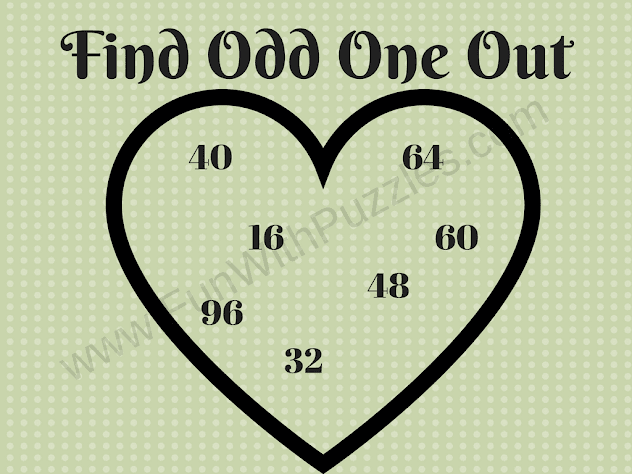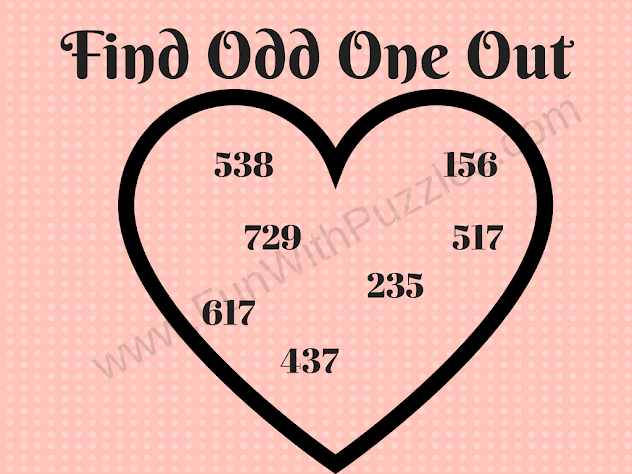Odd One Out Puzzles is a very interesting puzzle type. In these puzzles out of given few objects, one has to find out the odd object which does not follow a specific property that all other follows. The odd-one-out puzzles can be visual puzzles in which some pictures are given. Two out of these pictures are the same and one is a different one.
In these Odd One Out Puzzles, some numbers are given. All but one number follow one specific property which makes these numbers part of the group. However, there is only one number in each of the puzzles which does not follow this property and hence that number is the odd one out. Try finding this Odd Number in each of the puzzles given below.1. Out One Out Math Brain Teaser2. Out One Out Math Puzzle3. Out One Out  Riddle4. Out One Out Mind Twister Puzzle5. Out One Out Mind Cracking Puzzle

In these kinds of Visual Odd-One-Out puzzles earlier we have created Hut Odd One Out puzzles in which one has to find which Hut is different from others. Also, one can check out Mixed Odd One Out Picture puzzles which are tricky puzzles as it contains very tough-to-visualize differences. Kids can check some easy Spot the difference Puzzles in which one picture out of the given three pictures is different from the other two pictures. There are also some Mathematical Odd one-out puzzles that one should try before solving today's puzzles as this post also contains Math Odd one Out puzzles. Today's Odd One Out Puzzles are for kids, teens as well as adults. These puzzles start with the easy puzzles and keep getting tough as one proceeds.